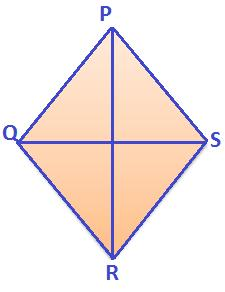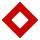# Diamond

Calculate the length of the two diagonals of the diamond if:

a = 13 cm
v = 12 cm

Correct result:

u1 =  21.63 cm
u2 =  14.42 cm

#### Solution:We would be pleased if you find an error in the word problem, spelling mistakes, or inaccuracies and send it to us. Thank you!Tips to related online calculators
Pythagorean theorem is the base for the right triangle calculator.

#### You need to know the following knowledge to solve this word math problem:

We encourage you to watch this tutorial video on this math problem:

## Next similar math problems:

• Diamond diagonalsCalculate the diamonds' diagonals lengths if the diamond area is 156 cm square and the side length is 13 cm.
• Rhombus and inscribed circleIt is given a rhombus with side a = 6 cm and the radius of the inscribed circle r = 2 cm. Calculate the length of its two diagonals.
• Rhombus diagonalsIn the rhombus ABCD are given the sizes of diagonals e = 24 cm; f = 10 cm. Calculate the side length of the diamond and the size of the angles, calculate the content of the diamond
• Rhombus and diagonalsThe lengths of the diamond diagonals are e = 48cm, f = 20cm. Calculate the length of its sides.
• Diamond diagonalsCalculate the diamond's diagonal lengths if its content is 156 cm2 and the side length is 13 cm.
• RhombusIt is given a rhombus of side length a = 19 cm. Touch points of inscribed circle divided his sides into sections a1 = 5 cm and a2 = 14 cm. Calculate the radius r of the circle and the length of the diagonals of the rhombus.
• DiagonalsGiven a rhombus ABCD with a diagonalsl length of 8 cm and 12 cm. Calculate the side length and content of the rhombus.
• Rhombus and diagonalsThe a rhombus area is 150 cm2 and the ratio of the diagonals is 3:4. Calculate the length of its height.
• DiamondSide length of diamond is 35 cm and the length of the diagonal is 56 cm. Calculate the height and length of the second diagonal.
• Diamond sideThe diagonals of the diamond are 18 cm and 14 cm long. Calculate the length of the diamond side.
• Diamond diagonalsFind the diamond diagonal's lengths if the area is 156 cm2 and side is 13 cm long.
• Rhombus baseCalculate the volume and surface area of prisms whose base is a rhombus with diagonals u1 = 12 cm and u2 = 10 cm. Prism height is twice base edge length.
• Rhombus IVCalculate the length of the diagonals of the rhombus, whose lengths are in the ratio 1: 2 and a rhombus side is 35 cm.
• KiteJohn a kite, which is diamond shaped. Its diagonals are 60 cm long and 90 cm long. Calculate: a) the diamond side b) how much paper John needs to make a kite if he needs paper on both sides and needs 5% of the paper for bending.
• Cuboid diagonalsThe cuboid has dimensions of 15, 20 and 40 cm. Calculate its volume and surface, the length of the body diagonal and the lengths of all three wall diagonals.
• Rectangle diagonalsIt is given rectangle with area 24 cm2 a circumference 20 cm. The length of one side is 2 cm larger than length of second side. Calculate the length of the diagonal. Length and width are yet expressed in natural numbers.
• DiagonalsA diagonal of a rhombus is 20 cm long. If it's one side is 26 cm find the length of the other diagonal.